Block Diagrams

# Block Diagrams - Notes | Study Control Systems - Electrical Engineering (EE)

 Table of contentsWhat is a Block Diagram?Elements of Block DiagramRules for Block Diagram ReductionExample of Block Diagram Reduction1 Crore+ students have signed up on EduRev. Have you?

Any system can be described by a set of differential equations, or it can be represented by the schematic diagram that contains all the components and their connections. However, these methods do not work for complicated systems. Block diagram comes in role for such complicated systems.

What is a Block Diagram?

Block diagrams consist of a single block or a combination of blocks. These are used to represent the control systems in pictorial form.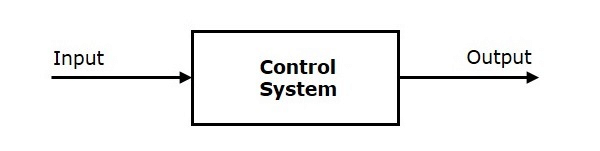For representing any system using block diagram, it is necessary to find the transfer function of the system which is the ratio of Laplace of output to Laplace of input.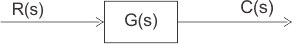Where,

R(s) = Input

C(s) = output

G(s) = transfer function

Then, the system can be represented as:

C(s) = R(s).G(s)

How the Block Diagram Representation Simplifies the System?

• So basically, the block diagram of a system is generated so that one can apply the transfer function approach.
• By doing so, the transfer function of each element can be determined and thus the system can be analyzed in a simple manner.
• Thus, in a simple term, we can say that a block diagram is the pictorial representation of a system in which the different elements of the system are sequentially connected.
• This sequential arrangement of the elements allows the easy determination of the relationship between cause and effect that exists between input and output of the system.

Block Diagram Algebra in Control Systems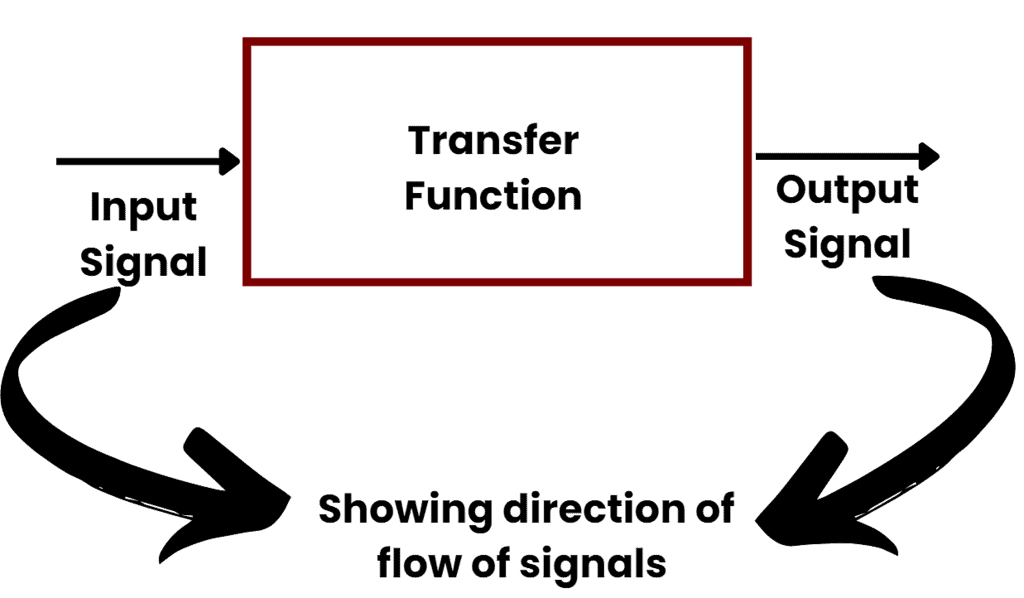• The functional block or simply block is a symbol for the mathematical operation on the input signal to the block that produces the output.
• The transfer functions of the components are usually entered in the corresponding blocks, which are connected by arrows to indicate the direction of the flow of signals.

Note: Sometimes, block diagrams become complex for some of the control systems and hence evaluation of their performance become difficult. For that, we require simplification (or reduction) of the block diagram which is carried out by block diagram rearrangement.

The various points for analyzing and reduction of these units are explained below:

Elements of Block Diagram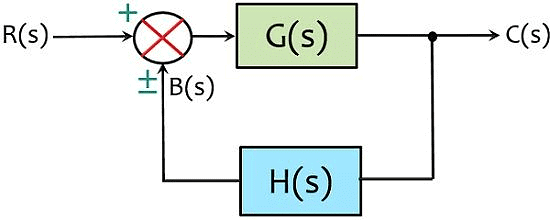A block diagram

Functional Block

• The functional block represents the elements or components of the control system.
• Basically, in block diagram representation, each element of the control system is represented by a block. This block is the functional block.
• Thus, we can say that a specific block represents the mathematical operation performed by that element on the input in order to achieve the desired output.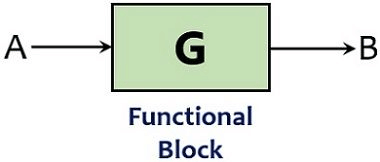The transfer function of the block shows the mathematical function of the element present as a block.

The elements used for the connectivity in a block diagram are given as follows.

1. Summing Point

It refers to the summing operation on the incoming signals. The point in the block diagram representation where the multiple signals are compared is known as the summing point.

(i) Summing Point Representation in a Block Diagram: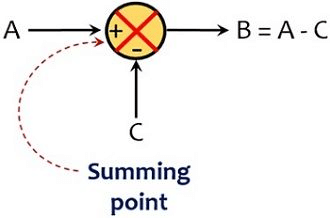It should be noted that the dimensions of the incoming signals on which the summing operation is performed, must be same.

2. Take-off Point

In a closed-loop system, part of the output is fed back to the input that acts as feedback for the system. So, the take-off point is that point from where a portion of the signal is taken as feedback for the system.

(i) Take-off Point Representation in a Block Diagram: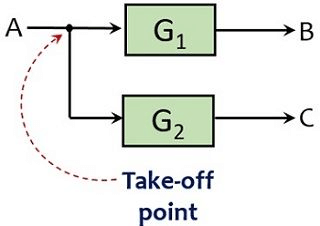When a number of blocks are connected in block diagram then overall transfer function can be obtained by block diagram reduction technique.

Rules for Block Diagram Reduction

1. Blocks in Cascade Reduction (Serially connected blocks)

• When blocks are connected in series then the overall transfer function of all the blocks is the multiplication of the transfer function of each separate block in the connection.

Suppose we have two blocks in cascade connection as shown below: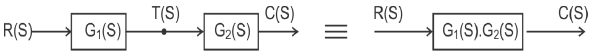We can calculate: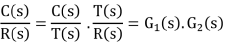• Thus, we can replace two blocks with different transfer functions into a single one having the transfer function equal to multiplication of each transfer function without altering the output.

Question for Block Diagrams
Try yourself:The overall transfer function from block diagram reduction for cascaded blocks is :

2. Blocks in Parallel Reduction

• In case the blocks are connected parallely then the transfer function of the whole system will be the addition of the transfer function of each block (considering sign).

Suppose two blocks are connected parallely as given below: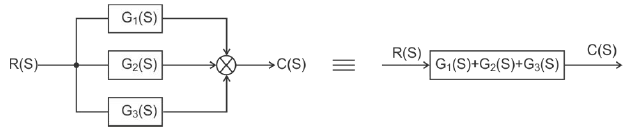• So, two parallely connected blocks can be replaced by a single block with a summation of the transfer function of each block.

Question for Block Diagrams
Try yourself:The overall transfer function of two blocks in parallel are :

3. Moving a Take-off Point After the Block

Suppose we have a combination of take-off point and block as shown below: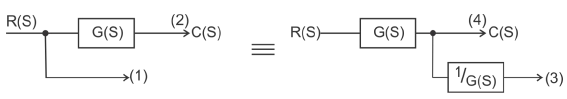• If we need to shift the take-off point ahead of the block, then we must keep ‘(1)’ as it is.

Here (1) = R(s)

• So, even after shifting, (1) must be R(s) and for this, we have to add a block with gain which is reciprocal of the gain of the originally present block.
• As the actual gain is G(s) so the additional block will have a gain of 1/G(s). Hence R(s) will remain the same: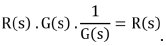4. Moving a Take-off Point Before the Block

Suppose there is a take-off point ahead of block as given below: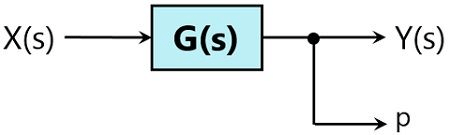• In order to move the take-off point behind the block, we need to keep the value of ‘p’ same. Here p = X(s)G(s).
• But with backward movement p will become X(s). So, we have to add another block with the same gain as the original gain. This will make the value of p = X(s)G(s)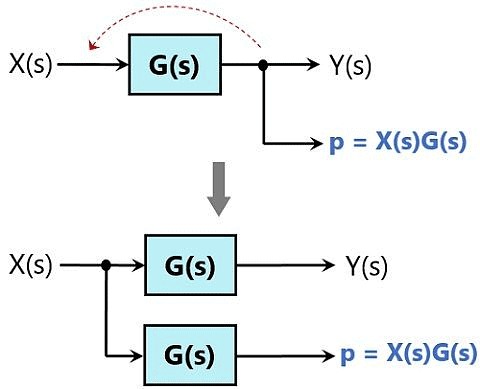5. Moving a Summing Point After the Block

Let's Suppose we have a configuration of summing point and block as given below: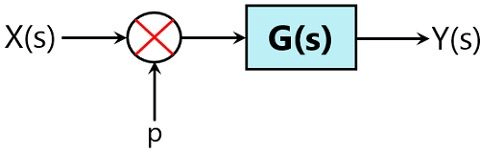• If the summing point is to be moved from backward to forward of the block, then Y(s) will become X(s)G(s)+p. However, earlier Y(s) was [X(s)+p]G(s).
• So, to have unaltered output, we need to add a block having exact gain as the original one.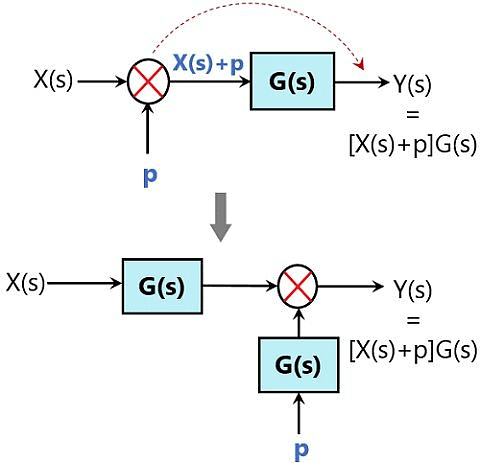6. Moving a Summing Point Before the Block

Suppose we have a combination where we have a summing point present after the block as shown below:

•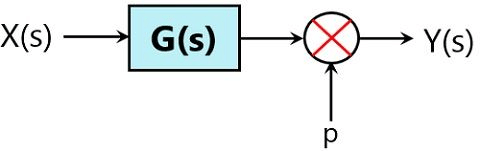We need to move this summing point behind the position of the block without changing the response.
• So, for this, a block with gain which is reciprocal of the actual gain is to be inserted in the configuration in series.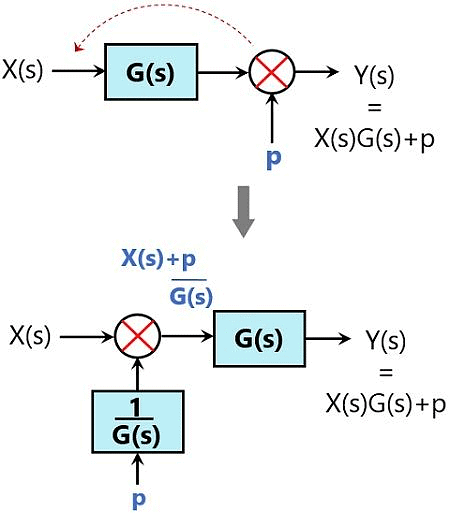7. Interchanging Two Summing Points

Consider a combination of two summing points directly connected with each other as shown below: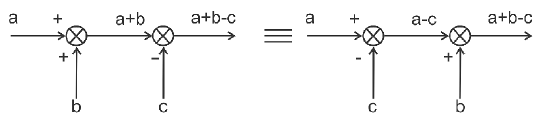We can use associative property and can interchange these directly connected summing points without altering the output.

8. Moving a Take-Off Point Before a Summing Point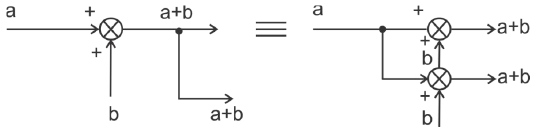9. Moving a Take-Off Point After a Summing Point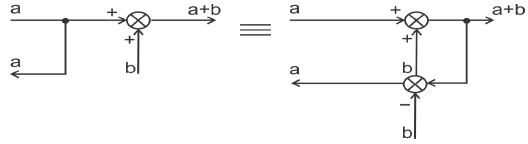10. Splitting/ Combining the Summing Point

• A summing point having 3 inputs can be split into a configuration having 2 summing points with separated inputs without disturbing the output. Or three summing points can be combined to form a single summing point with the consideration of each given input.

The figure below shows the above-discussed configuration: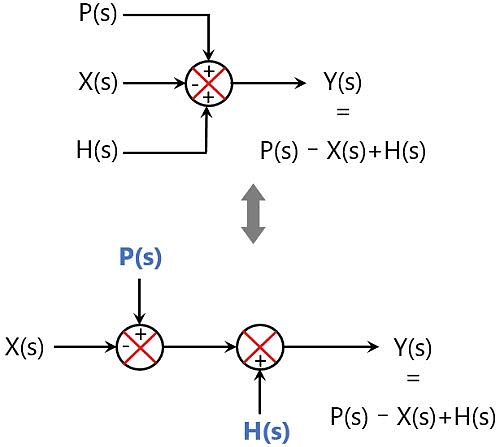11. Eliminating Feedback Loop

We have already derived in our previous article that the gain of a closed-loop system with positive feedback is defined as: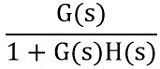So to remove the feedback loop, feedback gain must be used.

Therefore, we can have: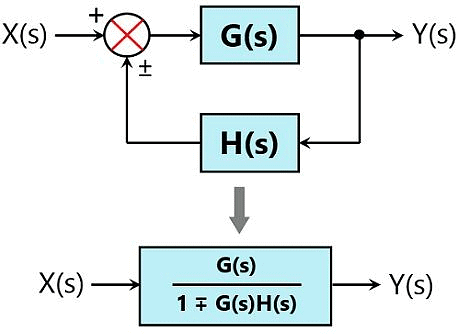Example of Block Diagram Reduction

Till now we have seen the important rules to be kept in mind while reducing the block diagram. Let us now see an example to have a better understanding of the same.

First, see the procedural steps to be followed for solving block diagram reduction problems:

1. The directly connected blocks in series must be reduced to a single block.
2. Further, reduce the parallely connected block into a single block.
3. Now reduce the internally connected minor feedback loops.
4. If shifting does not increase the complexity, then try having the take-off point towards the right while summing point towards left.
5. Repeat the above-discussed steps to have a simplified system.
6. Now determine the transfer function of the overall closed-loop simplified system.

Q. Consider a closed-loop system shown here and find the transfer function of the system: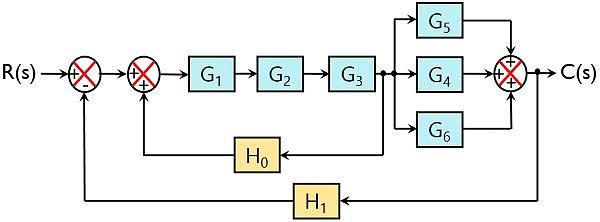Solution:

Reducing the 3 directly connected blocks in series into a single block, we will have: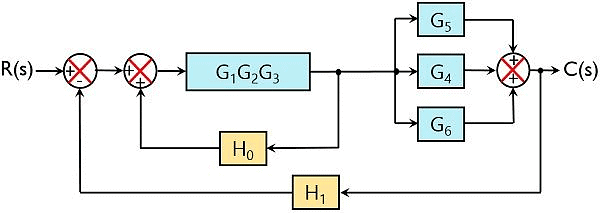Further, we can see 3 blocks are present that are connected parallely. Thus on reducing blocks in parallel, we will have: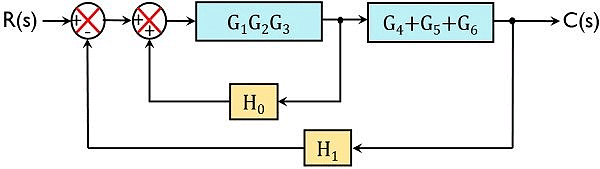Further on simplifying the internal closed-loop system, the overall internal gain will be: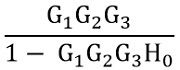So, we will have: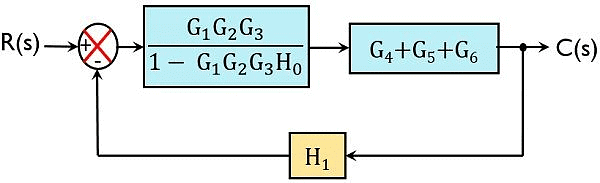Now reducing the two blocks in series: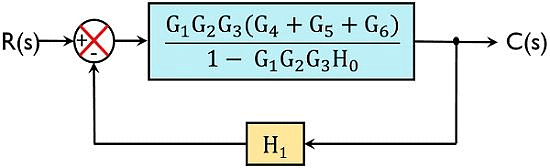So, this is the reduced canonical form of a closed-loop system.

We know gain of the closed-loop system is given as: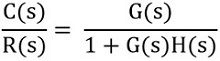Therefore,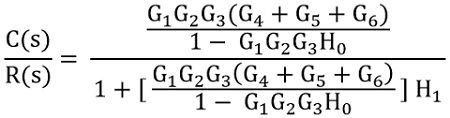On simplifying the equation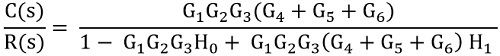This is the overall transfer function of the given control system.

The document Block Diagrams - Notes | Study Control Systems - Electrical Engineering (EE) is a part of the Electrical Engineering (EE) Course Control Systems.
All you need of Electrical Engineering (EE) at this link: Electrical Engineering (EE)

54 docs|45 tests

## Control Systems

54 docs|45 tests

Track your progress, build streaks, highlight & save important lessons and more!

,

,

,

,

,

,

,

,

,

,

,

,

,

,

,

,

,

,

,

,

,

;